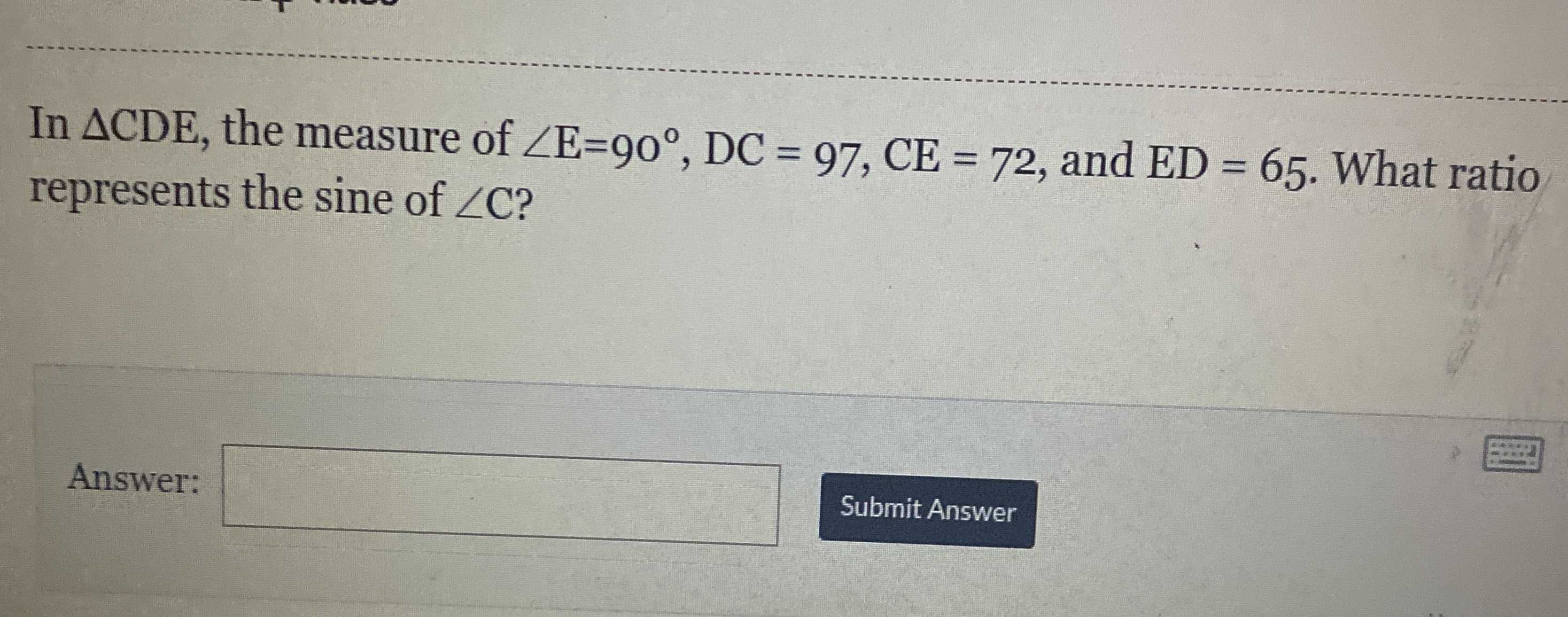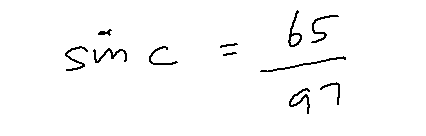### ¿Todavía tienes preguntas de matemáticas?

Pregunte a nuestros tutores expertos
Trigonometry
PreguntaIn $$\triangle CDE$$ , the measure of $$\angle E = 90 ^ { \circ } , DC = 97 , CE = 72$$ , and $$ED = 65 .$$ What ratio represents the sine of $$\angle C$$ ?